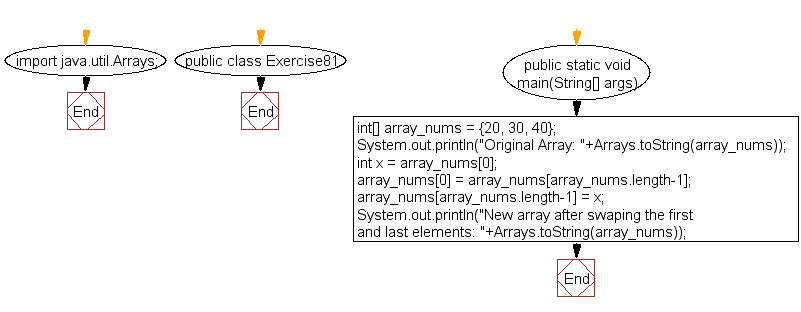﻿ Java exercises: Swap the first and last elements of an array and create a new array - w3resource# Java Exercises: Swap the first and last elements of an array and create a new array

## Java Basic: Exercise-81 with Solution

Write a Java program to swap the first and last elements of an array (length must be at least 1) and create a new array.

Sample Solution:

Java Code:

``````import java.util.Arrays;
public class Exercise81 {
public static void main(String[] args)
{
int[] array_nums = {20, 30, 40};
System.out.println("Original Array: "+Arrays.toString(array_nums));
int x = array_nums;
array_nums = array_nums[array_nums.length-1];
array_nums[array_nums.length-1] = x;
System.out.println("New array after swaping the first and last elements: "+Arrays.toString(array_nums));
}
}
```
```

Sample Output:

```Original Array: [20, 30, 40]
New array after swaping the first and last elements: [40, 30, 20]
```

Flowchart:Java Code Editor: